• python分段函数编写
千次阅读
2020-11-20 21:48:24

对于学编程的人来说，能将编写的程序封装成函数是一件多么开心的事，Python中也有大量的函数封装，那在Python怎么编写分段式函数呢？下面小编教给大家一些方法。

工具/原料

笔记本电脑（以Win7系统为例）；

开发Python的软件（如：PyCharm）。

方法/步骤

1

绘制分段函数：y=4sin(4πt)-sgn(t-0.3)-sgn(0.72-t)

代码：

#!/usr/bin/python

# -*- coding:utf-8 -*-

import numpy as np

import matplotlib.pyplot as plt

#绘制分段函数：y=4sin(4πt)-sgn(t-0.3)-sgn(0.72-t)

def sgn(x):

if x > 0:

return 1

elif x < 0:

return -1

else:

return 0

t = np.arange(0, 1, 0.01)

y = []

for i in t:

y_1 = 4 * np.sin(4 * np.pi * i) - sgn(i - 0.3) - sgn(0.72 - i)

y.append(y_1)

plt.plot(t, y)

plt.xlabel("t")

plt.ylabel("y")

plt.title("Heavsine")

plt.show()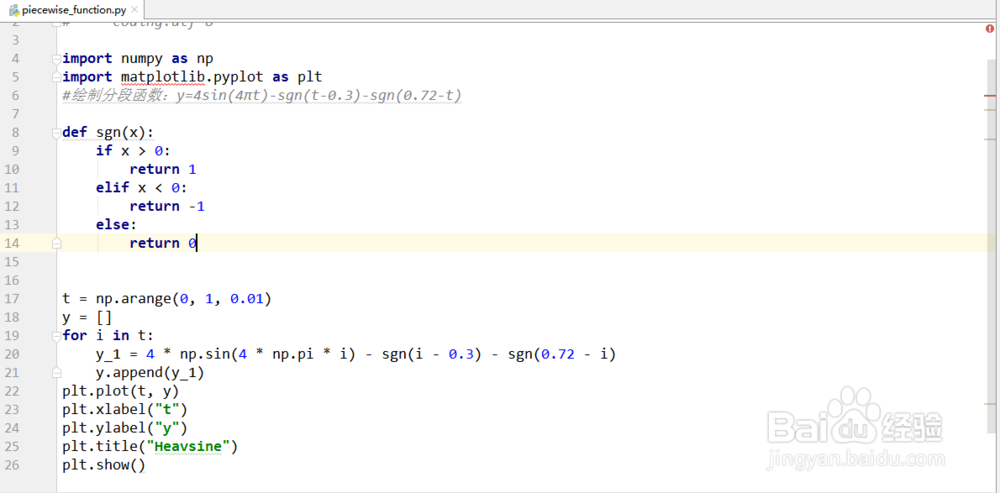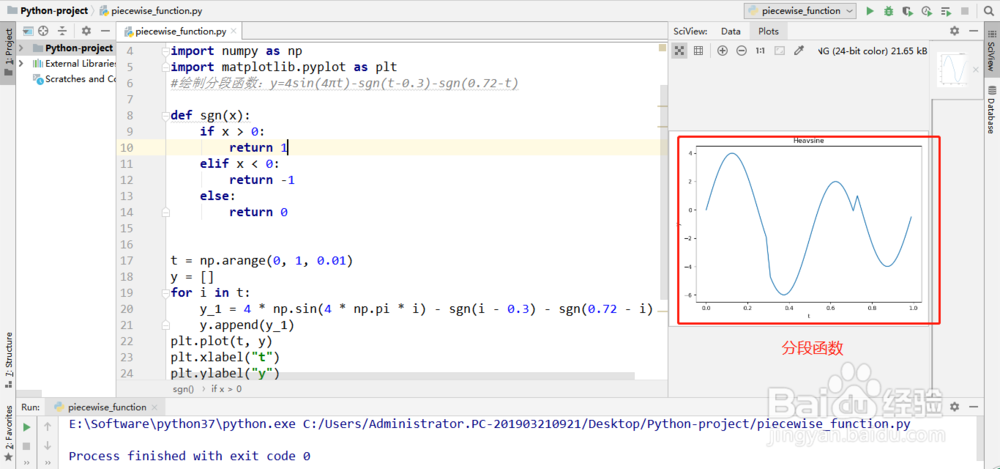2

使用Matplotlib绘制分段函数：

代码：

#!/usr/bin/python

# -*- coding:utf-8 -*-

import numpy as np

import matplotlib.pyplot as plt

def sgn(value):

if value < 4:

return 20

else:

return 15

plt.figure(figsize=(6, 4))

x = np.linspace(0, 8, 100)

y = np.array([])

for v in x:

y = np.append(y, np.linspace(sgn(v), sgn(v), 1))

l = plt.plot(x, y, 'b', label='type')

plt.legend()

plt.show()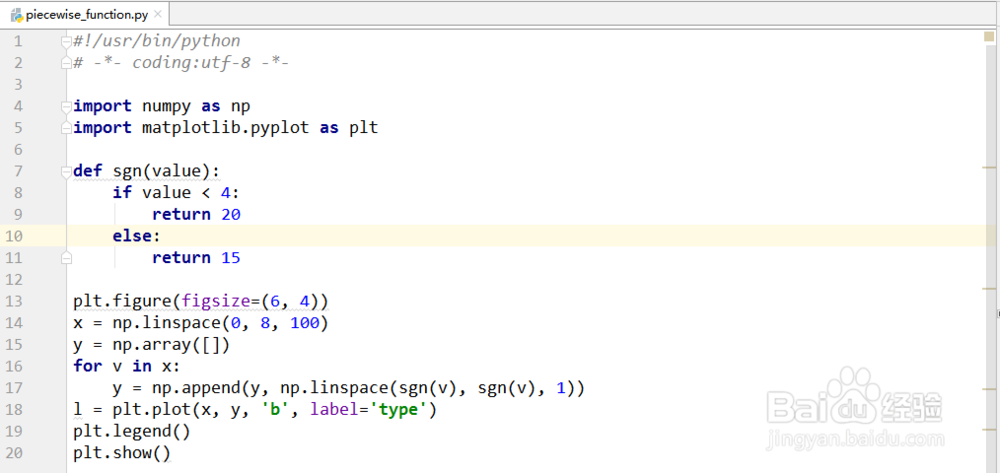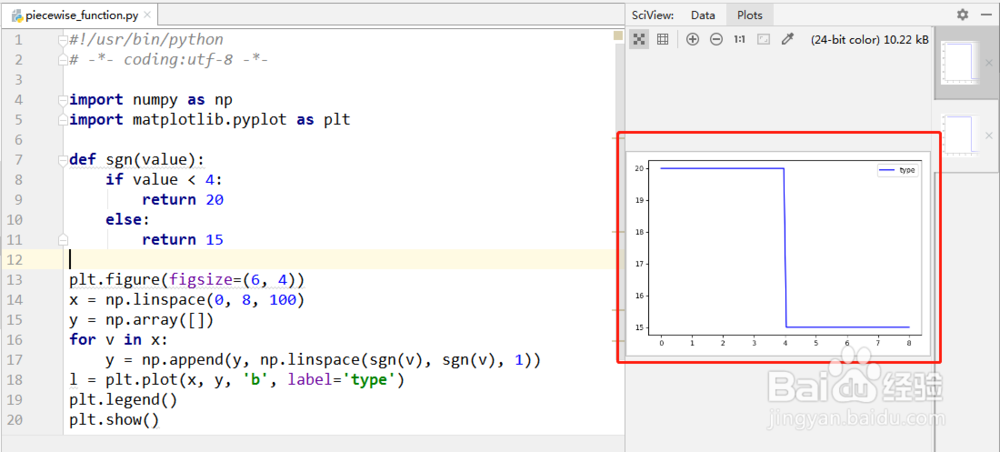3

绘制三角波形：

#!/usr/bin/python

# -*- coding:utf-8 -*-

import numpy as np

import matplotlib.pyplot as plt

def triangle_wave(x, c, c0, hc):

x = x - int(x) #三角波周期为1 因此只取小数部分进行计算

if x < c0:

return x / c0 * hc

elif x >= c:

return 0.0

else:

return (c-x)/(c-c0)*hc

x = np.linspace(0, 2, 1000)

y = np.array([triangle_wave(t, 0.6, 0.4, 1.0) for t in x])

plt.figure()

plt.plot(x, y)

plt.ylim(-0.2, 1.2) #限制y的范围

plt.show()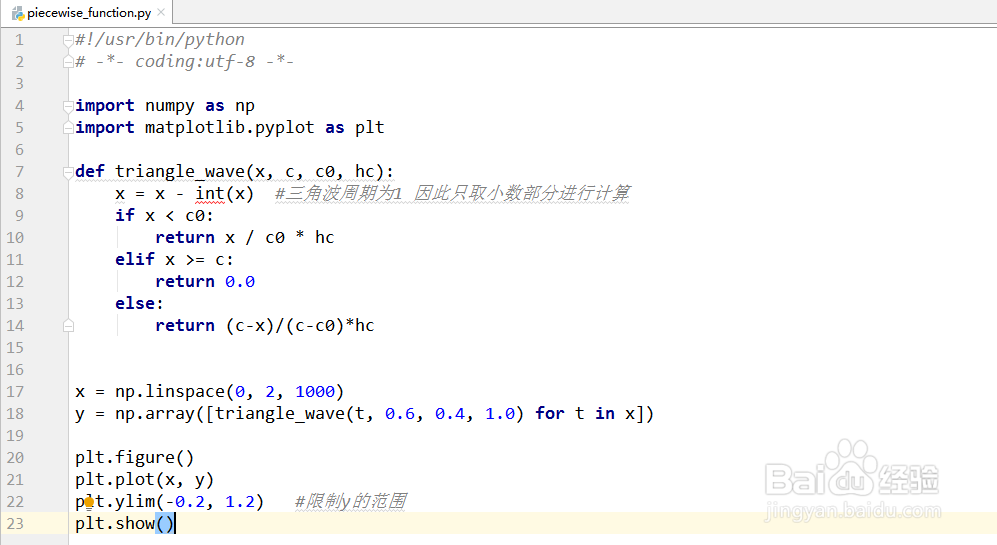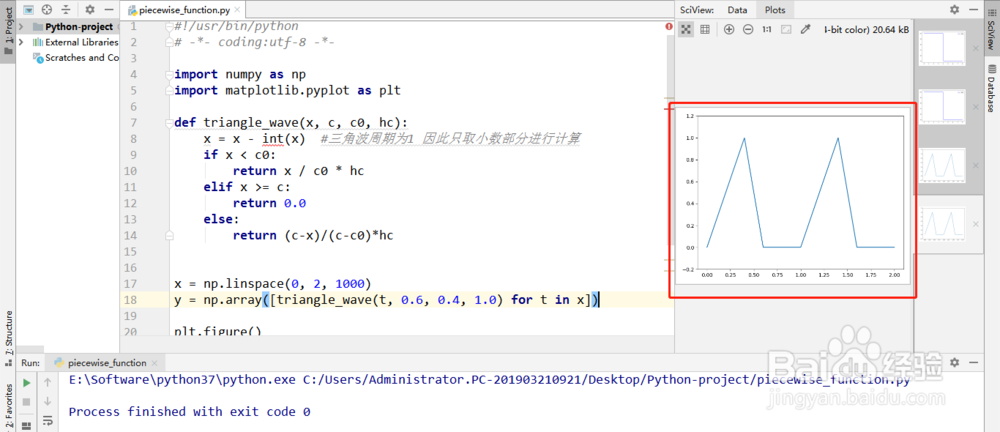END

注意事项

上面实例均是在Python的开发软件PyCharm中编写的，点击运行后是后面的效果，其它的Python运行环境也可以（基于Python3最好）；

使用matplotlib模块之前使用pip命令安装一下：pip install matplotlib

经验内容仅供参考，如果您需解决具体问题(尤其法律、医学等领域)，建议您详细咨询相关领域专业人士。

举报作者声明：本篇经验系本人依照真实经历原创，未经许可，谢绝转载。

展开阅读全部

更多相关内容
• C语言自定义函数(图文详解)_后端开发每个C程序都至少有一个函数，即主函数main()，在实际开发中...python分段函数如何编写？python编写分段函数的方法：1.绘制分段函数：y=4sin(4πt)-sgn(t-0.3)-sgn(0.72-t)代码：...

C语言自定义函数(图文详解)_后端开发

每个C程序都至少有一个函数，即主函数main()，在实际开发中，程序的任务往往比较复杂，如果全部的代码都写在main()函数中，main()函数将非常庞大臃肿，结构复杂，代码重复。下面我们就来看看自定义函数。python分段函数如何编写？

python编写分段函数的方法：

1.绘制分段函数：y=4sin(4πt)-sgn(t-0.3)-sgn(0.72-t)

代码：

#!/usr/bin/python

# -*- coding:utf-8 -*-

import numpy as np

import matplotlib.pyplot as plt

#绘制分段函数：y=4sin(4πt)-sgn(t-0.3)-sgn(0.72-t)

def sgn(x):

if x > 0:

return 1

elif x < 0:

return -1

else:

return 0

t = np.arange(0, 1, 0.01)

y = []

for i in t:

y_1 = 4 * np.sin(4 * np.pi * i) - sgn(i - 0.3) - sgn(0.72 - i)

y.append(y_1)

plt.plot(t, y)

plt.xlabel("t")

plt.ylabel("y")

plt.title("Heavsine")

plt.show()2.使用Matplotlib绘制分段函数：

几款好用的C语言编译器推荐_后端开发

一些刚开始接触C语言编译的小伙伴想下载一款C语言编译器来使用，网络上有不少C语言编译器相关的软件，让人很难抉择。那么，C语言编译器哪个好？下面就让我们来看看吧。

代码：

#!/usr/bin/python

# -*- coding:utf-8 -*-

import numpy as np

import matplotlib.pyplot as plt

def sgn(value):

if value < 4:

return 20

else:

return 15

plt.figure(figsize=(6, 4))

x = np.linspace(0, 8, 100)

y = np.array([])

for v in x:

y = np.append(y, np.linspace(sgn(v), sgn(v), 1))

l = plt.plot(x, y, 'b', label='type')

plt.legend()

plt.show()3.绘制三角波形：

#!/usr/bin/python

# -*- coding:utf-8 -*-

import numpy as np

import matplotlib.pyplot as plt

def triangle_wave(x, c, c0, hc):

x = x - int(x) #三角波周期为1 因此只取小数部分进行计算

if x < c0:

return x / c0 * hc

elif x >= c:

return 0.0

else:

return (c-x)/(c-c0)*hc

x = np.linspace(0, 2, 1000)

y = np.array([triangle_wave(t, 0.6, 0.4, 1.0) for t in x])

plt.figure()

plt.plot(x, y)

plt.ylim(-0.2, 1.2) #限制y的范围

plt.show()推荐教程：《python视频教程》

展开全文• python分段函数如何编写？python编写分段函数的方法：1.绘制分段函数：y=4sin(4πt)-sgn(t-0.3)-sgn(0.72-t)代码：#!/usr/bin/python# -*- coding:utf-8 -*-import numpy as npimport matplotlib.pyplot as plt#绘制...python分段函数如何编写？

python编写分段函数的方法：

1.绘制分段函数：y=4sin(4πt)-sgn(t-0.3)-sgn(0.72-t)

代码：#!/usr/bin/python

# -*- coding:utf-8 -*-

import numpy as np

import matplotlib.pyplot as plt

#绘制分段函数：y=4sin(4πt)-sgn(t-0.3)-sgn(0.72-t)

def sgn(x):

if x > 0:

return 1

elif x < 0:

return -1

else:

return 0

t = np.arange(0, 1, 0.01)

y = []

for i in t:

y_1 = 4 * np.sin(4 * np.pi * i) - sgn(i - 0.3) - sgn(0.72 - i)

y.append(y_1)

plt.plot(t, y)

plt.xlabel("t")

plt.ylabel("y")

plt.title("Heavsine")

plt.show()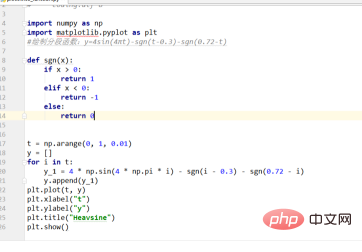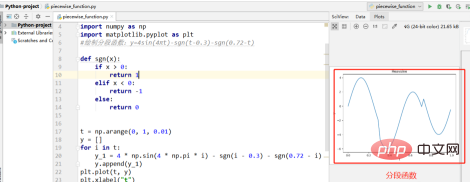2.使用Matplotlib绘制分段函数：

代码：#!/usr/bin/python

# -*- coding:utf-8 -*-

import numpy as np

import matplotlib.pyplot as plt

def sgn(value):

if value < 4:

return 20

else:

return 15

plt.figure(figsize=(6, 4))

x = np.linspace(0, 8, 100)

y = np.array([])

for v in x:

y = np.append(y, np.linspace(sgn(v), sgn(v), 1))

l = plt.plot(x, y, 'b', label='type')

plt.legend()

plt.show()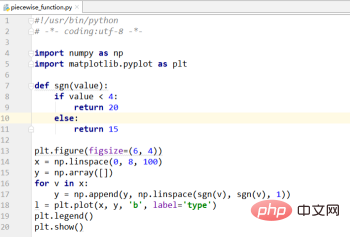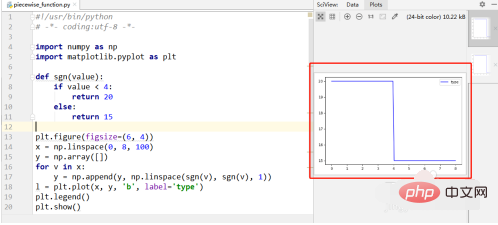3.绘制三角波形：#!/usr/bin/python

# -*- coding:utf-8 -*-

import numpy as np

import matplotlib.pyplot as plt

def triangle_wave(x, c, c0, hc):

x = x - int(x) #三角波周期为1 因此只取小数部分进行计算

if x < c0:

return x / c0 * hc

elif x >= c:

return 0.0

else:

return (c-x)/(c-c0)*hc

x = np.linspace(0, 2, 1000)

y = np.array([triangle_wave(t, 0.6, 0.4, 1.0) for t in x])

plt.figure()

plt.plot(x, y)

plt.ylim(-0.2, 1.2) #限制y的范围

plt.show()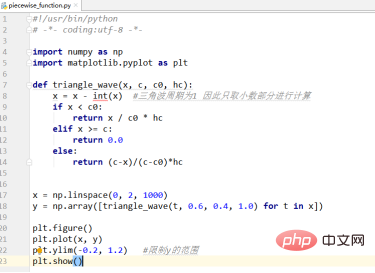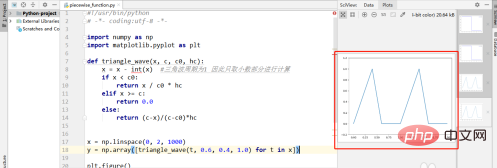展开全文• ## python分段函数

千次阅读 2020-11-20 21:48:23
广告关闭腾讯云11.11云上盛惠 ，精选热门产品助力上云，...segmentation fault (core dumped) python脚本: #! usrbinenv python import ctypesimport osimport sys # linux machine codebuf +=xbdxfcxa1x5dx63xd9xe...广告关闭

腾讯云11.11云上盛惠 ，精选热门产品助力上云，云服务器首年88元起，买的越多返的越多，最高返5000元！

segmentation fault (core dumped) python脚本: #! usrbinenv python import ctypesimport osimport sys # linux machine codebuf +=xbdxfcxa1x5dx63xd9xeexd9x74x24xf4x5ex31buf +=xc9xb1x1cx31x6ex14x03x6ex14x83xeexfcx1ebuf +=x54x37x1ex86x0ex7axe7x8fx31x6bxe8xefxb8buf +=x68x8ex6ex59x6exbfxbdx1ex5exe4xcaxf...

生成器函数在执行的时候，默认不会执行函数体，会返回生成器3.yield：相当于return可以返回数据，但是yield不会彻底中断函数，会分段执行函数 #例子：不...在python种有三种方式来获生成器 1. 通过生成器函数 2. 通过各种推导式来实现生成器 3. 通过数据的转换也可以获取生成器生成器函数1. 函数中如果由yield...插值法又称“内插法”，是利用函数f(x)在某区间中已知的若干点的函数值，作出适当的特定函数，在区间的其他点上用这特定函数的值作为函数f (x)的近似值。 常用的插值方法有lagrange插值、newton插值、分段插值、hermite插值、样条插值等等。 这里我们就介绍一下最常用到的lagrange、newton、分段插值法及python实现...

生成器 生成器的本质就是迭代器 在python中有三种方式来获取生成器:1. 通过生成器函数 2. 通过各种推导式来实现成器 3. 通过数据的转换也可以获取生成器 生成器的特点和迭代器一样. 取值方式和迭代器一样(__next__(), send(): 给上一个yield传值). 生成器一般由生成器函数或者生成器表达式来创建 其实就是手写的迭代...打开一个shp图层的表，选择需要计算的字段，选中字段计算器（arcgis10.3 中文版为例）：在开头处可以选择vb或者python，中部左是字段选择，右边则是函数...这里需要简单说明的是，系统预设的函数有时候并不能满足我们的需求，例如：当你需要对这个字段的数据进行分段，预设函数就无法满足了。 这个自定义的函数...

byte表示字节序列python2中为正常显示中文，引入coding声明，python3中不需要python2中是raw_input()函数，python3中是input()函数7.xrange和range的区别？ range:根据start与stop指定的范围以及step设定的步长，生成一个序列。 xrange:用法与range完全相同，所不同的是生成的不是一个数组，而是一个生成器...2、如何在一个函数内部修改全局变量函数内部global声明 修改全局变量? 3、列出5个python标准库os：提供了不少与操作系统相关联的函数 sys:通常用于命令行参数re: 正则匹配math: 数学运算datetime:处理日期时间4、字典如何删除键和合并两个字典del和update方法 ? 5、谈下python的gilgil 是python的全局解释器锁，同一...python语言特性1 python的函数参数传递看两个例子：? 所有的变量都可以理解是内存中一个对象的“引用”，或者，也可以看似c中void*的感觉。 这里记住的是类型是属于对象的，而不是变量。 而对象有两种，“可更改”（mutable）与“不可更改”（immutable）对象。 在python中，strings， tuples， 和numbers是不可更改...

可以使用类的__dict__字典属性或python内置的dir()函数来获取类的属性 ? 实例属性 实例仅拥有数据属性(严格意义上来说，方法是类属性)通常通过构造器...这些特殊方法是python中用来扩充类的强大工具，它们可以实现 模拟标准类型 重载操作符特殊方法允许类通过重载标准操作符+，*，甚至包括分段下标及映射操作...三、字符串操作1、len()在python里有很多函数，可以对字符串进行操作：len()是看字符串长度的。 print(len(小小哆啦))4当我们使用unicode的时候，按中文字符的个数显示，但使用python2.x里的默认字符编码，就会是三倍的数值。 因为python3开始，默认字符编码是unicode，所以在python3.x里是没有问题的。 2、合并字符...案例5、编写一个冒泡排序函数需求：冒泡排序法是一种经典的排序算法模型，请使用python语言编写一个冒泡排序算法的函数，并能支持任意的一维序列对象实现...（2）理解了分段求和问题，解决办法就是：根据业绩覆盖的范围，分段计算，再相加。 （3）判断业绩覆盖的范围，肯定是用分支语句啦。 根据销售额计算奖金源...

(1分段)alist = adict = {} adict = 1 b = adict if 1:print(sheng xin bao dian great!) 32 + 32 alist = alist.add(4) alist =join(alist) int(a) 3 0 for i in range(10) print(l) sheng xin * 3 type =1python文件读写函数open的mode参数中r, w, a, t, b, x分别是什么意思？ (3分)python中如何获取当前所在的工作...

pd.dataframe()生成结果结果：esproc? python? 3. 字段分段题目介绍：库表data有两个字段，id和anomoalies，数据如下：? 我们的目的是将anomoalies字段按空格拆分为多个字符串，每个字符串和原id字段形成新的记录。 esproc? a4：news函数的用法在第一例中已经解释过，这里不再赘述。 python：import time import ...

guido von rossum开始写python语言的编译器。 1991年2月：第一个python编译器（同时也是解释器）诞生，它是用c语言实现的（后面又出现了java和c#实现的版本jython和ironpython，以及pypy、brython、pyston等其他实现），可以调用c语言的库函数。 在最早的版本中，python已经提供了对“类”，“函数”，“异常处理”等...

定义析构函数 pass del saysomething(self):定义方法(成员函数) print self.voice tom = animal()tom.saysomething() jerry = animal(hello) jerry.saysomething()类的特殊属性可以使用类的__dict__字典属性或python内置的dir()函数来获取类的属性dir(myclass) myclass.__dict__每个对象自己的__dict__属性中保存了本...而super()可用于传入实例或类型对象，语法super(type)运算符重载运算重载是指在方法中拦截内置的操作——当类的实例出现在内置操作中，python会自动调用自定义的方法，并且返回自定义方法的操作结果运算符重载让类拦截常规的python运算类可重载所有python表达式运算符类也可重载打印、函数调用、属性点号运算等内置...

辣么，飞机的飞行曲线函数，要怎么表达，我就有点懵逼，想半天没想到牛逼的方法，就用最通俗的分段函数硬杠了出来（4条线段+4个点）然后就是把这一张张的图片，按顺序合成一个gif，代码如下：#! usrbinpython3.6# -*- coding: utf-8 -*-# __author__: ed frey# date:201895 import imageio,os def create_gif(image...

多个文档在一个yaml文件，使用 --- 分隔方式来分段如：yaml文件中数据# 分段yaml文件中多个文档---animal1: dogage: 2---animal2: catage:32.python脚本读取一个yaml文件中多个文档方法python获取yaml数据时需使用load_all函数来解析全部的文档，再从中读取对象中的数据# yaml文件中含有多个文档时，分别获取文档中...2、如何在一个函数内部修改全局变量函数内部global声明 修改全局变量? 3、列出5个python标准库os：提供了不少与操作系统相关联的函数 sys:通常用于命令行参数re: 正则匹配math: 数学运算datetime:处理日期时间4、字典如何删除键和合并两个字典del和update方法 ? 5、谈下python的gilgil 是python的全局解释器锁，同一...

展开全文• ## 通过Python绘制分段函数

万次阅读 多人点赞 2020-06-17 12:47:16
如何利用python绘制分段函数 下面通过整理的两个例子对两种方法进行说明： 例子1 主要思路是将函数y在各区间的值表示出来。 首先导包 from matplotlib import pyplot as plt import numpy as np 其次，确定定义域...python
• ## Python 计算分段函数

千次阅读 多人点赞 2021-04-24 18:24:49
计算分段函数 题目描述: 计算下列分段函数f(x)的值： 公式 输入格式: 输入在一行中给出实数x。 输出格式: 在一行中按“f(x) = result”的格式输出，其中x与result都保留一位小数。 输入样例1: 10 输出样例1: f...
• 我正在尝试将分段定义的函数适合Python中的数据集.我现在已经搜索了很长一段时间,但我还没有找到答案是否有可能.要了解我想要做的事情,请查看以下示例(这对我不起作用).在这里,我试图将移位的绝对值函数(f(x)= | x-p...
• 本文将要为您介绍的是Python练习题2.2计算分段函数,教程操作步骤:本题目要求计算下列分段函数f(x)的值：输入格式:输入在一行中给出实数x。输出格式:在一行中按“f(x) = result”的格式输出，其中x与result都保留一位...
• Python分段函数值的方法：首先打开python编辑器；然后使用【If...else..】进行判断，代码为【x = eval(input()) y = 0 if x=1 and x
• 方便起见，在这里使用一个分段函数的例子：计算三角波形（例子取自张若愚的《Python科学计算》）三角波形具有周期性，因此我们只需要考虑0~1之间的这个范围就可以了，在这个范围里，又分了三个不同的表达式。...
• x=eval(input("请输入一个x:")) if x<0 or x>=20: y=0 elif 0<x<5: y=x elif 5<x<10: y=3*x-5 else: y=0.5*x-2 print(y)python
• 分段函数 本题目要求计算下列分段函数f(x)的值（x为从键盘输入的一个任意实数）： 输入格式: 输入在一行中给出实数 x。 输出格式: 在一行中按“f(x)=result”的格式输出，其中x与result都保留两位小数。 输入样例: ...python
• python分段函数怎样编写？python编写分段函数的要领：1.绘制分段函数：y=4sin(4πt)-sgn(t-0.3)-sgn(0.72-t)代码：#!/usr/bin/python# -*- coding:utf-8 -*-import numpy as npimport matplotlib.pyplot as plt#绘制...
• 本博文源于python科学计算，旨在解析如何使用python进行计算分段函数值。下面就以复杂的二元函数进行演示。分段函数值配合定义域是这段代码的亮点，以后写代码时更应该多多揣摩。python
• 编写程序，计算下列分段函数y=f(x)的值。 y=-x+2.5; 0 <= x < 5 y=2-1.5(x-3)(x-3); 5 <= x < 10 y=x/2-1.5; 10 <= x < 20 输入 一个浮点数N,0 <= N < 20 输出 输出N对应的分段函...python
• ## Python绘制分段函数

千次阅读 2020-11-20 18:28:50
绘制分段函数：y=4sin(4πt)-sgn(t-0.3)-sgn(0.72-t) import numpy as npimport matplotlib.pyplot as plt#绘制分段函数：y=4sin(4πt)-sgn(t-0.3)-sgn(0.72-t)def sgn(x)...09327相关推荐2019-09-28 17:10 −首先...
• C语言编程题 有一分段函数如下，要求用scanf函数输入整数x的值，求y值并在屏幕上输出。 有分段函数如下，编程实现输入整www.zhiqu.org 时间： 2020-11-22#include int main(){ int x; double y; scanf("%d",&x)...
• Description：计算下列分段函数f(x)的值： f(x) x 1/x x ≠ 0 0 x = 0 Input：输入仅一行，输入一个实数x。 Output：输出仅一行，按“f(x) = result”的格式输出，其中x与result都保留一位小数。 Sample...python
• 本题目要求根据以下分段函数的定义，计算输入的浮点数x对应的y值，输出结果保留两位小数。注意：使用math库python
• pytorch下可训练分段函数的写法 这篇博客主要讲如何写一个可训练求导的分段函数，并通过代码验证其可行性 假设我们要实现这样一个分段函数： F（a）={+1a>2.50.25∗a+0.3750.5<a≤2.5a−0.5<a≤0.50.25∗...python pytorch
• Spicy Chicken Code PTA Python 2.2Python
• 编程计算分段函数,根据键盘输入的x值,在屏幕输出y值.#include#includevoidmain(){floatx,y;scanf("%f",&a计算分段函数的值.根据输入的x值计算下列表达式中y的值.是c语言程序设计题.#includeintmain(){\x09floatx...
• 这一讲主要分段插值中的分段线性插值和分段Hermite插值，并给出分段插值的Python程序。在此之前需要注意一下，n为区间数，n+1为插值节点的个数。分段线性插值分段线性插值，需要两个列表，一个用于存放各点的x坐标，...
• 请帮我看一下这道编程题如何编写代码，编程计算分段函数分段函数如图，输入x的值，输出函数y的值 需要运用python,谢谢。python
• 中国大学MOOC: 编程计算下面的分段函数，根据从键盘输入的x值，在屏幕上输出y值。答：else if (x == 0)具有明显方向性和足够强度，用来确定画面主调和气氛的是：答：主光法律和道德是维护社会秩序的两种基本手段，......